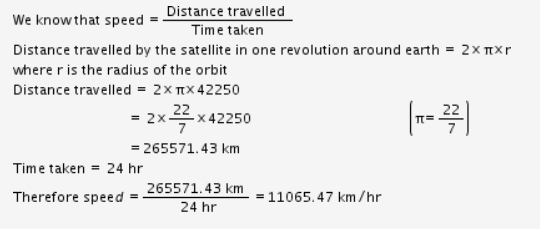# A satellite is moving in circular orbit of radius 42250 km. Calculate its speed

A satellite is moving in circular orbit of radius 42250 km. Calculate its speed if it takes 24 hour to revolve around the earth.

Radius of the circular orbit of along which the satellite is moving = 42250 km.
Time taken by the satellite to complete one revolution around earth = 24 h.The speed of the satellite = 11065.47 km/hr